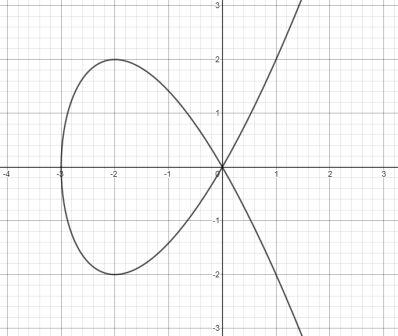# The curve with equation { y^2 = x^2(x + 3) } is called Tschirnhausen cubic. If you graph this...

## Question:

The curve with equation {eq}y^2 = x^2(x + 3) {/eq} is called Tschirnhausen cubic. If you graph this curve you will see that part of the curve forms a loop. Find the area enclosed by the loop.

## Definite Integral

This problem is based on the application of definite integral. It is preferable to draw the graph of the given equation so that it gets easier to visualize the problem. Using the concept of integration and by setting the limits of integration we will calculate the area of the curve.

The Tschirnhausen curve can be shown as:The equation of the curve is,

{eq}\displaystyle y^2=x^2(x+3) {/eq}

{eq}\displaystyle y=\pm\sqrt{x^2(x+3)} {/eq}

Taking {eq}x^2 {/eq} out of the square root, we can write it like,

{eq}\displaystyle y=|x|\sqrt{(x+3)} {/eq}

To make the area positive we will consider a negative sign else we will have just the lower half.

{eq}\displaystyle y=-x\sqrt{(x+3)} {/eq}

Since, it is symmetric about the {eq}x {/eq}-axis we will double the area so that we also calculate the upper half.

So, the area of curve can be given as,

{eq}\displaystyle A=2\int_{-3}^{0}-x\sqrt{x+3}dx {/eq}

Making the substitution,

{eq}u=x+3 {/eq}

{eq}du=dx {/eq}

And the limits of integration will change accordingly,

{eq}\displaystyle A=-2\int_{0}^{3}(u-3)\sqrt{u}du {/eq}

{eq}\displaystyle A=-2\int_{0}^{3}(u^{\frac{5}{2}}-3u^{\frac{1}{2}})du {/eq}

{eq}\displaystyle A=-2\left [ \frac{2}{5}u^{\frac{5}{2}}-3.\frac{2}{3}u^{\frac{3}{2}} \right ]_{0}^{3} {/eq}

Simplifying the integral we get,

{eq}\displaystyle A=\frac{-36\sqrt 3}{5}+12\sqrt 3 {/eq}

Thus, the area of the closed loop is:

{eq}\displaystyle \boxed{\displaystyle A=\frac{24\sqrt 3}{5}} {/eq}